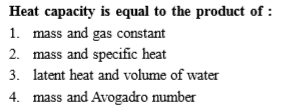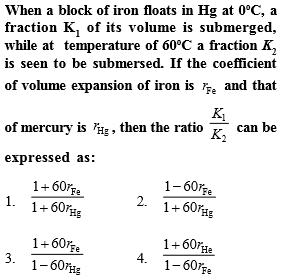# NEETprep Bank NEET Physics Thermal Properties of Matter Questions Solved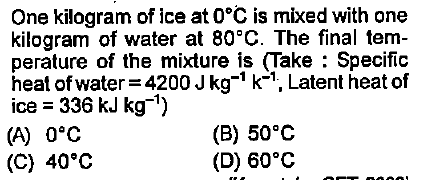Complete Question Bank + Test Series
Complete Question Bank

Difficulty Level: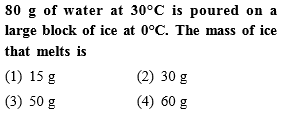Complete Question Bank + Test Series
Complete Question Bank

Difficulty Level: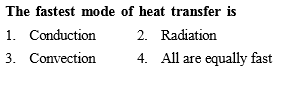Complete Question Bank + Test Series
Complete Question Bank

Difficulty Level: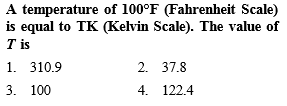Complete Question Bank + Test Series
Complete Question Bank

Difficulty Level: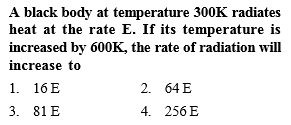Complete Question Bank + Test Series
Complete Question Bank

Difficulty Level: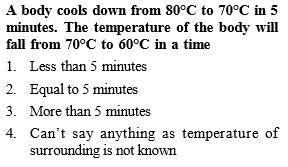Complete Question Bank + Test Series
Complete Question Bank

Difficulty Level: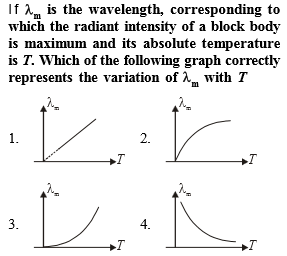Complete Question Bank + Test Series
Complete Question Bank

Difficulty Level:

If 48 gm of ${\mathrm{O}}_{3}$ at $27°\mathrm{C}$ is mixed with 64 gm of ${\mathrm{O}}_{2}$ at $327°\mathrm{C}$ in an adiabatic vessel as shown in the figure, then the final temperature of the mixture will be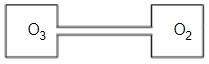1.  $200°\mathrm{C}$

2.  $227°\mathrm{C}$

3.  $314.5°\mathrm{C}$

4.  $235.5°\mathrm{C}$

Complete Question Bank + Test Series
Complete Question Bank

Difficulty Level: